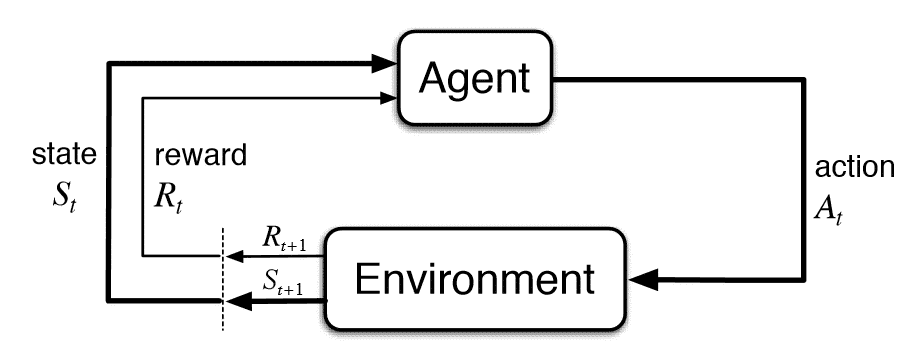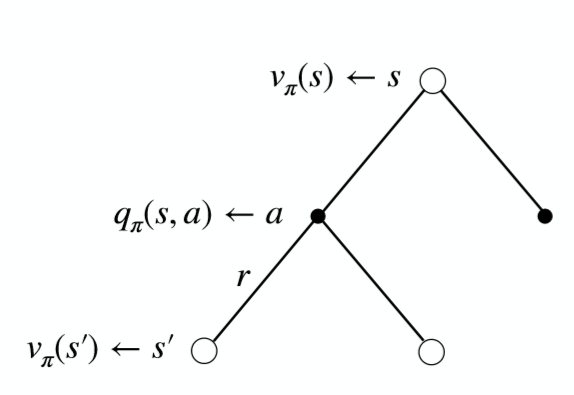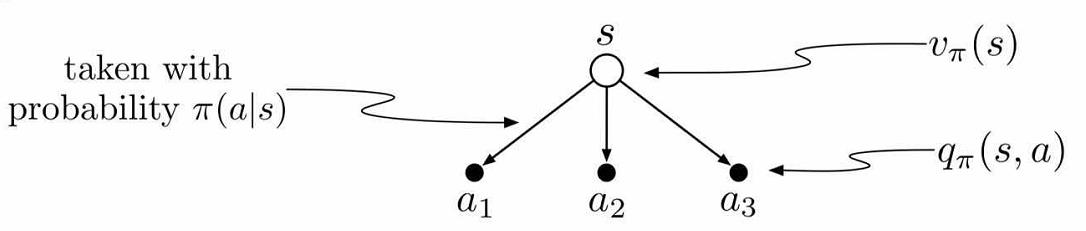# 0x1 强化学习简介

• 以reward作为监督信号
• 反馈具有延后性(不是及时的)
• 处理的数据为序列数据而非独立同分布# 0x2 MDP简介

RL是建立在​环境具有马尔科夫性的假设上的，即下一个状态的产生只取决于当前的状态，而与之前的状态无关。即

$P[S_{t+1}|S_t] = \mathbb P[S_{t+1}|S_1,...,S_t]$

​马尔可夫决策过程(Markov Decision Process, MDP)正式描述了强化学习的决策过程, MDP可以定义为<$S,A,P,R,\gamma$>, 分别表示state集合、action集合、状态转化概率、reward函数、衰减率。RL的policy有两种，确定性策略(deterministic policy)和随机性策略(stochastic policy)，前者表示对于一个策略$\pi$,给定状态$s$,策略会给出一个动作$a$, 记为$\pi(s)=a$。而后者对于一个策略$\pi$， 给定状态$s$, 会给出执行动作空间里的每个动作的概率分布, 记为$\pi(a|s)=\mathbb P[A_t=a|S_t=s]$, 在此，我们同时也假设了策略$\pi$也符合马尔可夫假设，即此时采取动作$a$的概率只和当前的状态$s$有关，而与其他要素无关。我们可以定义出环境转移的概率为

$P_{ss^{'}} = \mathbb P[S_{t+1} =s^{'}|S_t=s]$

$P_{ss^{'}}^a = \mathbb P[S_{t+1} =s^{'}|S_t=s,A_t=a]$

$P_{ss^{'}}^{\pi} = \sum_{a \in A} \pi(a|s)\mathbb P_{ss^{'}}^a$

$R_{s}^a = \mathbb E[R_{t+1} = r|S_t=s,A_t=a]$

$R_{s}^{\pi} = \sum_{a \in A} \pi(a|s)R_{s}^a$

$V_{\pi}(s) = \mathbb {E}_{\pi}[G_t|S_t=s]$

$Q_{\pi}(s,a) = \mathbb {E}_{\pi}[R_{t+1} + \gamma(V_{\pi}(S_{t+1}))|S_t=s, A_t=a]$

# 0x3 贝尔曼期望方程与最优方程## 状态值函数$V(s)$与动作值函数$Q(s,a)$的关系$V_{\pi}(s)=\sum_{a \in A} \pi(a|s) Q_{\pi}(s,a)$$Q_{\pi}(s,a)=R_{s}^a+\gamma \sum_{s^{'} \in S} P_{ss^{'}}^a V_{\pi}(s^{'})$

## 联系$V(s)$与$V(s^{’})$

$V_{\pi}=\sum_a \pi(a|s) (R_{s}^a+\gamma \sum_{s^{'} \in S} P_{ss^{'}}^a V_{\pi}(s^{'}))$

## 联系$Q(s,a)$与$Q(s^{’},a^{’})$

$Q_{\pi}(s,a)=R_{s}^a+\gamma \sum_{s^{'} \in S} P_{ss^{'}}^a \sum_{a^{'} \in A} \pi(a^{'}|s^{'}) Q_{\pi}(s^{'},a^{'})$

## 最优价值函数

$V^{*}(s)=\max_{\pi}V_{\pi}(s)$

$Q^{*}(s,a)=\max_{\pi}Q_{\pi}(s,a)$

## 贝尔曼最优方程

$V^{*}(s)=\max_{a} Q^{*}(s,a)$

$Q^{*}(s,a)=R_{s}^a+\gamma \sum_{s^{'} \in S} P_{ss^{'}}^a V^{*}(s^{'})$

$V^{*}(s)=\max_{a}(R_{s}^a+\gamma \sum_{s^{'} \in S} P_{ss^{'}}^a V^{*}(s^{'}))$

$Q^{*}(s,a)=R_{s}^a+\gamma \sum_{s^{'} \in S} P_{ss^{'}}^a \max_{a} Q^{*}(s^{'},a^{'})$

​如果我们完全直到环境信息，则问题可以转化为一个规划问题，通过动态规划求解，但大部分时候我们不知道转移概率、reward函数，不能直接通过贝尔曼方程求解。